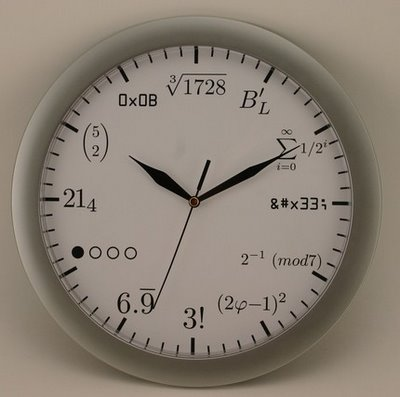A picture of nerd clock if you search on Google:Explanation of the twelve:

1. Legendre’s constant which says prime numbers appears at the rate of ln(n)
2. Infinite series 1+0.5+0.25+…
3. ASCII character “3” of code 0x33
4. Modular exponentiation
5. φ = Golden ratio constant, [1-sqrt(5)]/2
6. Factorial(3) = 6
7. 0.99999 = 1
8. Binary 0b1000
9. Base-4 number 21 = 2 $$\times$$ 4 + 1 = 9
10. Combination 5C2 = 10
11. Hex number 0xB = 11
12. Cubic root of 1728 = 12